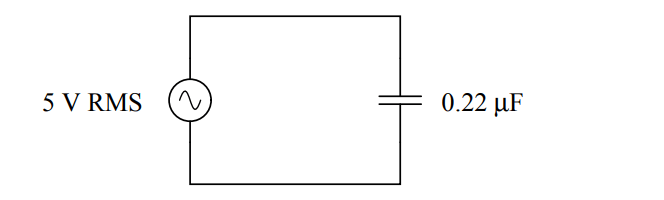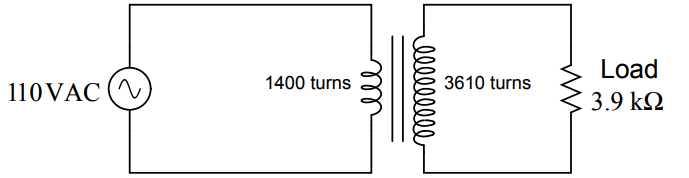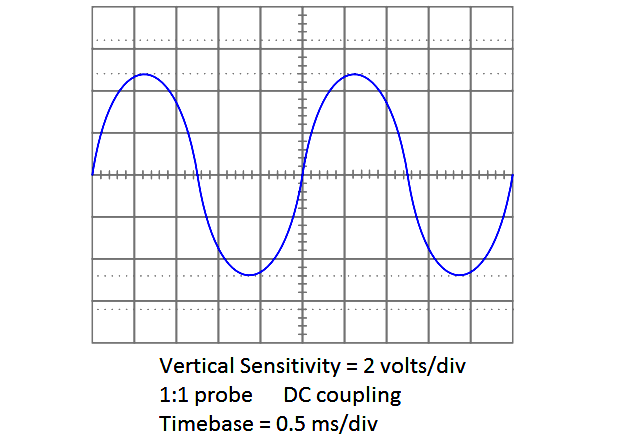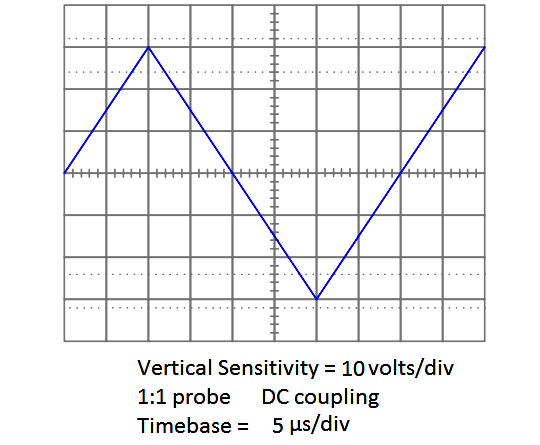# AC Circuits Multiple Choice Questions

AC Circuits Multiple Choice Questions

Question 1

What will happen to the current in this circuit as frequency increases?(A) Current will remain the same
(B) Cannot tell – not enough information given
(C) Current will decrease
(D) Current will decrease to a minimum and then increase
(E) Current will increase

Question 2

Resonance is defined as a condition in an AC circuit when:

(A) no more magnetic flux may be forced into an iron core
(B) opposition to electric current varies with frequency
(C) voltage and current waveforms are exactly 90o out of phase
(D) electrons are able to “tunnel” across an energy gap
(E) complementary reactances are equal and opposite.

Question 3

Calculate the source and load currents in this transformer circuit:(A) Isource = 77.22 mA Iload = 283.6 mA
(B) Isource = 187.5 mA Iload = 72.73 mA
(C) Isource = 186.5 mA Iload = 72.53 mA
(D) Isource = 164.2 mA Iload = 63.66 mA
(E) Isource = 72.53 mA Iload = 186.5 mA

Question 4

The function on an oscilloscope that “locks in” waveforms so that they do not scroll horizontally across the screen is called the.

(A) Horizontal sync
(B) Timebase
(C) Beam finder
(D) Trigger
(E) Cursor

Question 5

A voltage gain of 25 (expressed as a unitless ratio) may also be expressed as decibels (dB).

(A) 17.78
(B) 7.959
(C) 27.96
(D) 13.98
(E) 64.38

Question 6

In an AC circuit where current lags voltage by 35 deg, the power factor is:

(A) 0.819
(B) 0.574
(C) 0.700
(D) 1.414
(E) 0.707

Question 7

Determine the frequency of this waveform shown on an oscilloscope screen:(A) 250 Hz
(B) 0.4 Hz
(C) 10 kHz
(D) 10 Hz
(E) 400 Hz

Question 8

Calculate the RMS value of this triangle wave, as shown by the oscilloscope:(A) 17.31 volts
(B) 30 volts
(C) 3.2 volts
(D) 21.21 volts
(E) 32 volts

Question 9

If a communications cable has a characteristic impedance of 75 ohms, this means:

(A) The cable’s end-to-end resistance is 75 ohms
(B) The cable’s conductor-to-conductor resistance is 75 ohms
(C) The cable should be terminated with a resistance equal to 37.5 ohms
(D) The cable’s conductor resistance is 75 ohms per mile of length
(E) The cable appears to a pulse signal as a 75 ohm load

Question 10

A low pass filter is a circuit that performs the following function:

(A) Modifies the shape of an AC wave
(B) Blocks low-frequency signals from passing through
(C) Passes only the negative (low) portions of an AC wave
(D) Blocks high-frequency signals from passing through
(E) Attenuates low-frequency signals

Credits : by Tony R. Kuphaldt